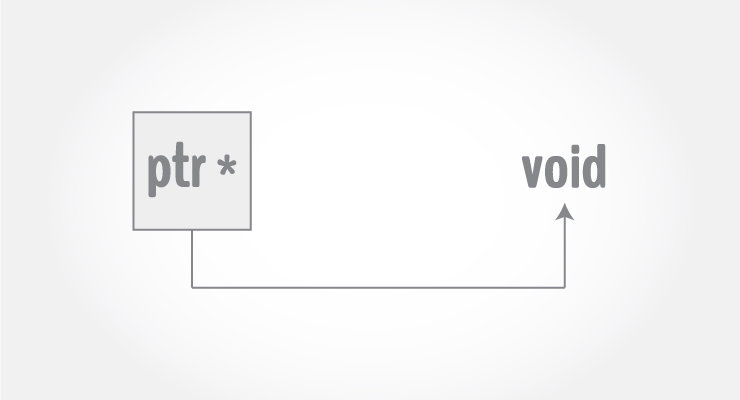# C++ Pointer to Void

In this article, you'll learn to use void pointers. These pointers can point to any type of data. This article will teach you to use them efficiently in your program.In C++, you cannot assign the address of variable of one type to a pointer of another type. Consider this example:

```int *ptr;
double d = 9;
ptr = &d;   // Error: can't assign double* to int*
```

However, there is an exception to this rule.

In C++, there is a general purpose pointer that can point to any type. This general purpose pointer is pointer to `void`.

```void *ptr; // pointer to void
```

## Example 1: C++ Pointer to Void

``````#include <iostream>
using namespace std;

int main() {
void* ptr;
float f = 2.3;
ptr = &f;     // float* to void

cout << &f << endl;
cout << ptr;

return 0;
}``````

Output

```0xffd117ac
0xffd117ac```

Here, the pointer `ptr` is given the value of `&f`.

The output shows that the void pointer ptr stores the address of a `float` variable f.